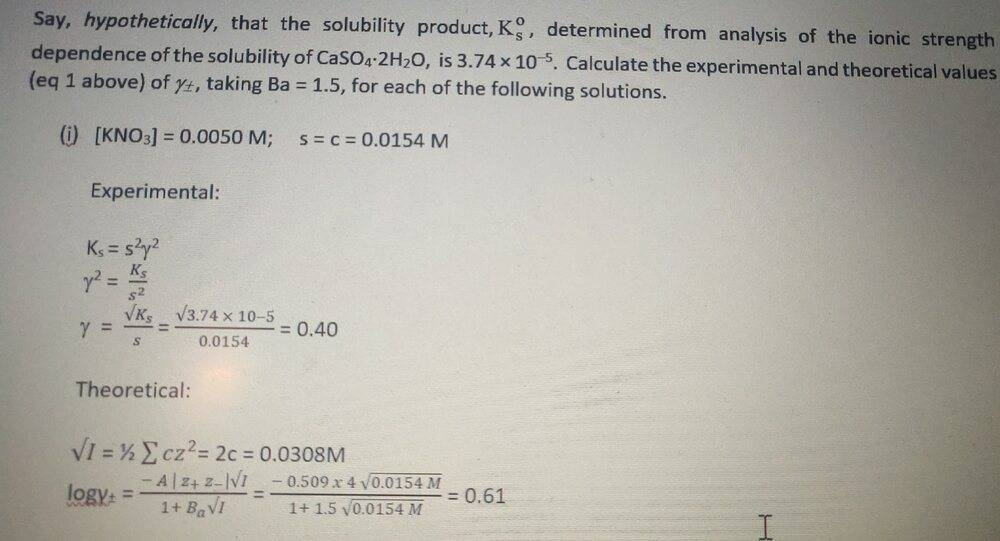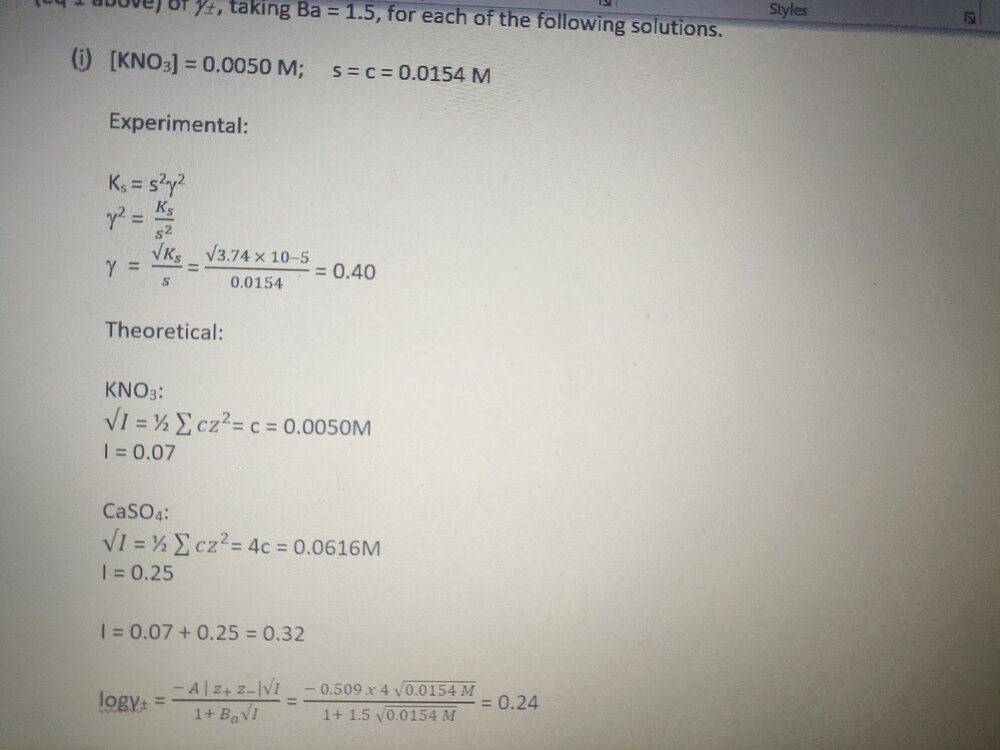# Please confirm my Activity Coefficient Calculations

• Chemistry
Yokoko
Homework Statement:
I've had a go at these but am unsure about the result. I thought the values would have to be closer to each other.
Relevant Equations:
See pictureHomework Helper
You have not calculated I correctly. I (not √I) = 1/2∑cz2 for ALL ions present.
Then you haven't used your calculated value for I in the equation for logγ. You used c. Why?
(Do it correctly, and it does come out close to the experimental value.)

YokokoThanks for your answer. Again, I'm not sure if I understood this correctly.

I used c as I thought s = c for simple ions. I now took the root of √I to get I.

I assumed the C for KNO3 = 0.0050 and C for CaSO4 = s = 0.0154

This might be where I am wrong?